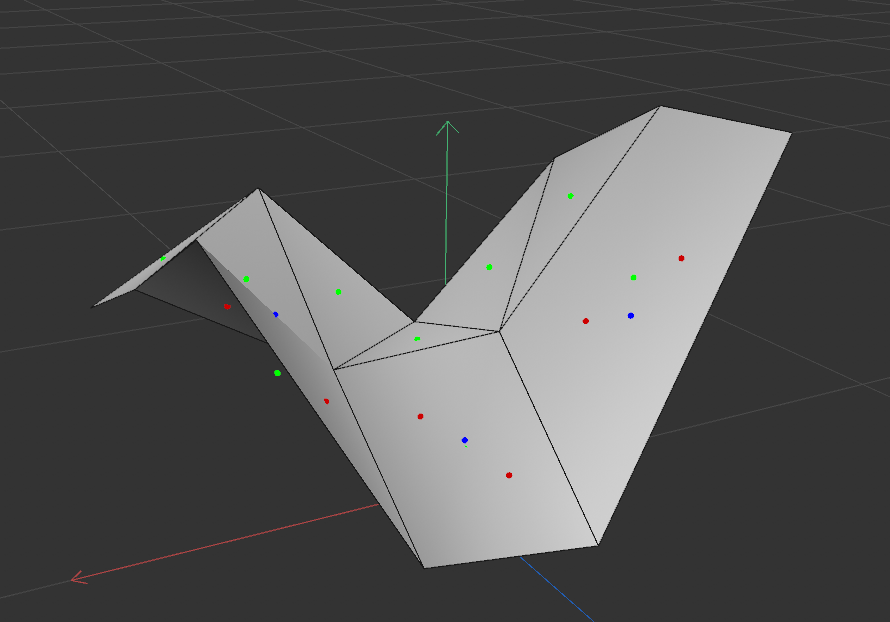# Get the "Position" of a Polygon

@ferdinand
I didn´t wanted to make a new thread. How to get the position of a polygon , when I have the selected polygon index?

Ah I got it for a quad.
adding the points positions and then divide by 4
(a + b + c + d) /4

but how to get it if it is a triangle?

edit: Forked from Remove points from a polygon
by @ferdinand

Cheers
Tom

Hello @ThomasB,

Thank you for reaching out to us. I have forked your thread because the question(s) of yours were disjunct from the topic of 'removing points from a polygon' of the original thread. Please also make sure to consolidate your question into one posting when you have follow-up questions or find a solution on your own before we answer. It was very manageable in this case, but it can be very distracting both for us and future readers when the initial topic of a thread is split over multiple postings with an unclear goal.

It depends a bit on what you mean by 'the position of a polygon'. The arithmetic mean of a triangle is just `(a + b + c) * (1./3.)`. Triangles are stored as quadrangles in case of `CPolygon`. A `CPolygon` which is a triangle returns `True` for `IsTriangle()` and evaluates as `True` for `CPolygon.c == CPolygon.d`.

But the arithmetic mean of the points of a quadrangle can lie outside of the planes of the two internal triangles when they are not coplanar. What users would then often consider to be the 'center' of the polygon is the midpoint of its edge AC. The dividing edge of the internal triangles of a quadrangle will lie for `CPolygon` always on the edge AC. Other than the arithmetic mean, the midpoint will always lie on 'the surface of the polygon'.

For triangles, the arithmetic mean will always lie in the plane defined by the triangle, but it can also make sense to compute the geometric (or harmonic) mean instead, depending on what you want to do.

Looking at geometry_polygonobject_s26.py might also help in this context.

Cheers,
Ferdinand

Result:The arithmetic mean point (green) for the quad in the foreground on the left lies 'below' the surface of the polygon, while the mean point (green) for the quad on the right lies 'above' it. The midpoint (blue) of the internal edge AC of both polygons lies on the surface of the polygon and is usually what users would consider the 'center' of the polygon. For the (almost) co-planar polygon in the middle, the mean point and AC midpoint are (almost) identical. For all quads: The arithmetic mean of their internal triangles in red. In the background in green, the arithmetic mean of direct triangle polygons.

File: poly.c4d

Code:

``````"""Demonstrates how to compute points that could be considered 'central' in a polygon.

Must be attached as Python Programming tag to a polygon object. Will draw the mean point in green,
and for quads the mid-point of AC in blue, as well as the mean of both internal triangles in red.

Python SDK - geometry_polygonobject_s26.py: https://tinyurl.com/2jtwzddn
"""

import c4d

op: c4d.BaseTag # The Python scripting tag

def main() -> None:
"""Not needed in this case.
"""
pass

def draw(bd: c4d.BaseDraw) -> bool:
"""Called by Cinema 4D to let the Python tag draw into the viewport #bd.
"""
# Get the object that tag is attached to.
obj: c4d.BaseObject =  op.GetMain()
if not isinstance(obj, c4d.PolygonObject) or obj.GetPolygonCount() < 1:
raise RuntimeError(f"{obj} is not a valid polygon object.")

# Get its points and polygons and pre-compute 1/3 and 1/4.
points: list[c4d.Vector] = obj.GetAllPoints()
polygons: list[c4d.CPolygon] = obj.GetAllPolygons()
fFourth: float = .25
fThird: float = 1./3.

# Set the drawing matrix to the polygon object, i.e., we now draw in the space the points are
# already in. Also set the point size of the drawn points.
bd.SetMatrix_Matrix(obj, obj.GetMg(), 5)
bd.SetPointSize(5)

# Iterate over all polygons.
for poly in polygons:
# Get the points of the polygon #poly. A triangle will simply repeat its third index, both
a, b, c, d = points[poly.a], points[poly.b], points[poly.c], points[poly.d]
isQuad: bool = not poly.IsTriangle() # equal to: poly.a != poly.d

# The list of points and colors we are going to draw.
dPoints: list[c4d.Vector] = []
colors: list[float] = []

# Compute the arithmetic mean of all points in the triangle or quadrangle. For quadrangles,
# this point might lie 'below' or 'above' the surface of the quadrangle (when its two
# internal triangles are not co-planar).
mean: c4d.Vector = (a + b + c + d) * fFourth if isQuad else (a + b + c) * fThird

dPoints.append(mean)
colors += [0., 1., 0.]

# In case of a quad, there are other 'interesting' points to find.
# The point users might consider to be the center of quad, the mid point of the
# dividing internal triangle edge. A CPolygon quadrangle is guaranteed to be
# triangulated over AC. Other than #mean, #center will always lie on the surface of the polygon.
center: c4d.Vector = (a + c) * .5

# The arithmetic mean of the two internal triangles. For triangles it might also be
# interesting to compute the geometric or harmonic mean of their points.
triMeanA: c4d.Vector = (a + b + c) * fThird
triMeanB: c4d.Vector = (a + c + d) * fThird

dPoints.append(center)
colors += [0., 0., 1.]
dPoints.append(triMeanA)
colors += [.8, 0., 0.]
dPoints.append(triMeanB)
colors += [.8, 0., 0.]

# Draw the points.
bd.DrawPoints(dPoints, colors, 3, None)

return True
``````

MAXON SDK Specialist
developers.maxon.net

@ferdinand
holythank you Ferndinand for this detailed description and your effort.

Cheers
Tom